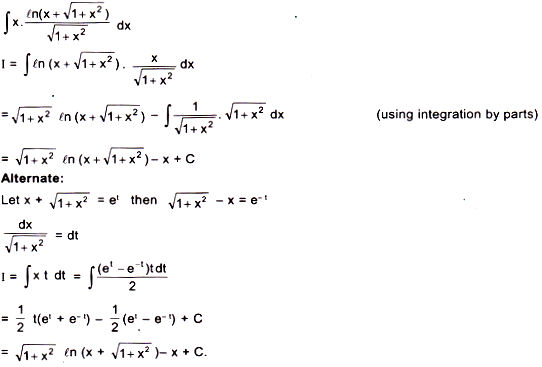Courses
Courses for Kids
Free study material
Free LIVE classes
MoreLIVE
Join Vedantu’s FREE Mastercalss

# The value of the expression $\int x.\frac{\ell n\left(x+\sqrt{1+{x}^{2}}\right)}{\sqrt{1+{x}^{2}}}dx$$\int {x.\frac{{\ell n\left( {x + \sqrt {1 + {x^2}} } \right)}}{{\sqrt {1 + {x^2}} }}dx}$ equals:Verified